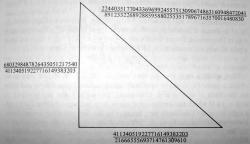The Simplest Rational Right Triangle with Area 157

# The Congruent Number Problem

[Up]

## Congruent Numbers

A positive integer n is a congruent number if it is the area of a right triangle with rational side lengths. The numbers 1, 2, 3, and 4 are not congruent, but 5, 6, and 7 are.

For any positive integer n, let En denote the elliptic curve defined by the equation y2 = x3 - n2x. Then n is a congruent number if and only if En has infinitely many solutions. The curves En are quadratic twists of the elliptic curve y2 = x3 - x, which is isogenous to the modular curve X0(32).